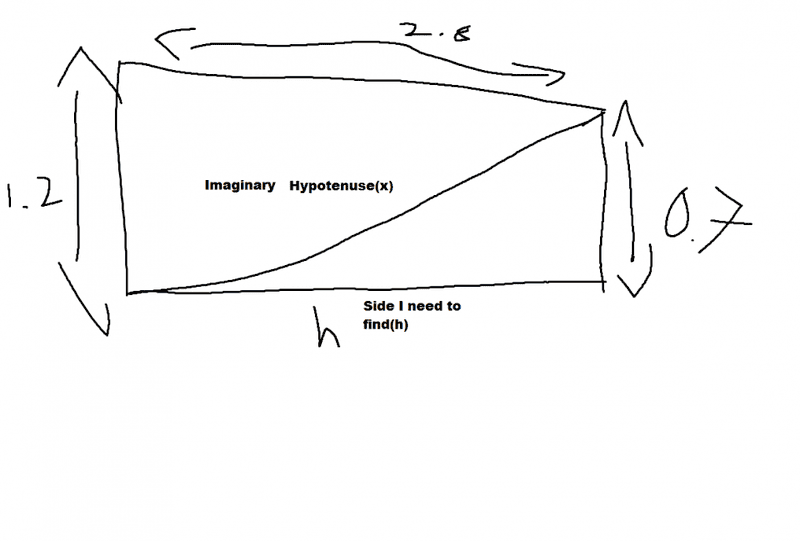# Basic Trig Problem

## Homework Statement

A loading ramp is 2.8m long. One end rests on a loading dock 0.7 meters above the ground, and the other end leads into the back of a a tractor trailer 1.2m above the ground. Find the horizontal distance between the back of the truck and the loading dock, to the nearest tenth of a meter.

a² + b² = c²

## The Attempt at a Solution

In order to solve this problem I figured out that you need to make an imaginary hypotenuse from the ground below the truck to the top of the loading dock. With that hypotenuse you need to figure out the horizontal distance between the back of the truck and the loading dock.
Attempt:
Let x represent the diagonal from the ground at the truck to the loading dock.
2.8^2 - .7^2 = x^2
√6.4 = √x^2
2.53m = x
That is for the imaginary hypotenuse. Now for the leg it asked for:
Let h represent the horizontal distance between the back of the truck to the start of the dock.
2.53^2 - .7^2 = h^2
6.4 - .49 = h^2
√5.91 = √h^2
2.43m = h
Then the therefor statement. The problem is, the textbook tells me the answer is 2.8. Where did I go wrong?
P.S
To get a mental image, just imagine a rectangle with a diagonal from the lower left corner to the upper right corner.

Last edited:

## Answers and Replies

fresh_42
Mentor
What do you need the ground and this imaginary hypotenuse for? Have you drawn the situation?

What do you need the ground and this imaginary hypotenuse for? Have you drawn the situation?
Yes, I have drawn it. You need the hypotenuse because the length it is asking me to solve requires it.
View attachment 101935Last edited by a moderator:
fresh_42
Mentor
So you want to find ##h##. But ##h## can also be found at another place. This should simplify your calculation. Maybe you see it better, if you only think of the truck, the ramp and the dock. You don't need the ground.

Edit: Your error is that you apply Pythagoras on a triangle which isn't a right one.

•Liam C
So you want to find ##h##. But ##h## can also be found at another place. This should simplify your calculation. Maybe you see it better, if you only think of the truck, the ramp and the dock. You don't need the ground.

Edit: Your error is that you apply Pythagoras on a triangle which isn't a right one.
Ohhh, okay I think I can solve it from here, thank you.